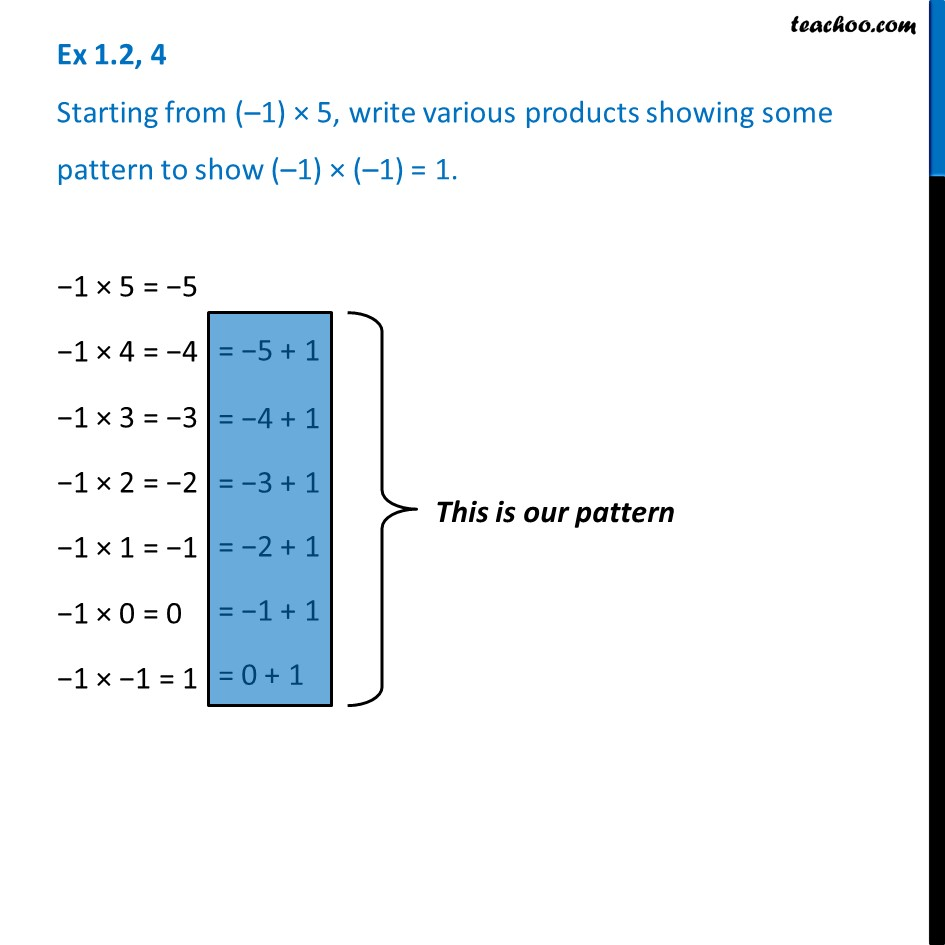Ex 1.2

Chapter 1 Class 7 Integers
Serial order wiseLearn in your speed, with individual attention - Teachoo Maths 1-on-1 Class

### Transcript

Ex 1.2, 4 Starting from (–1) × 5, write various products showing some pattern to show (–1) × (–1) = 1. −1 × 5 = −5 −1 × 4 = −4 −1 × 3 = −3 −1 × 2 = −2 −1 × 1 = −1 −1 × 0 = 0 −1 × −1 = 1